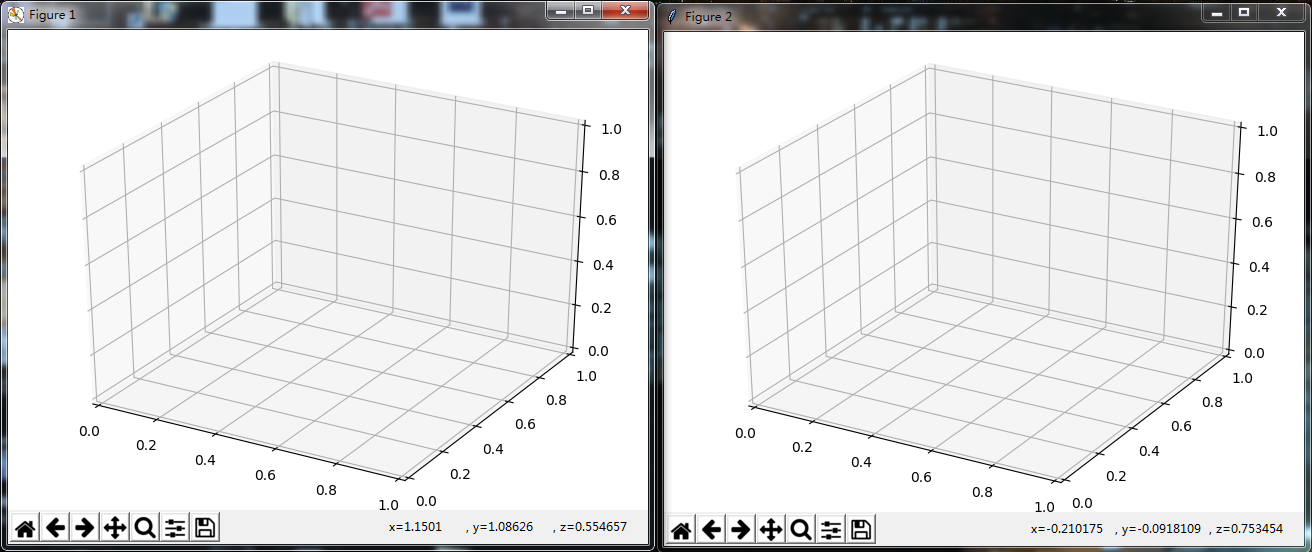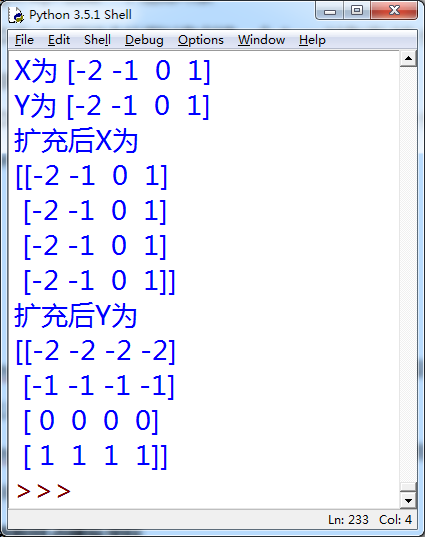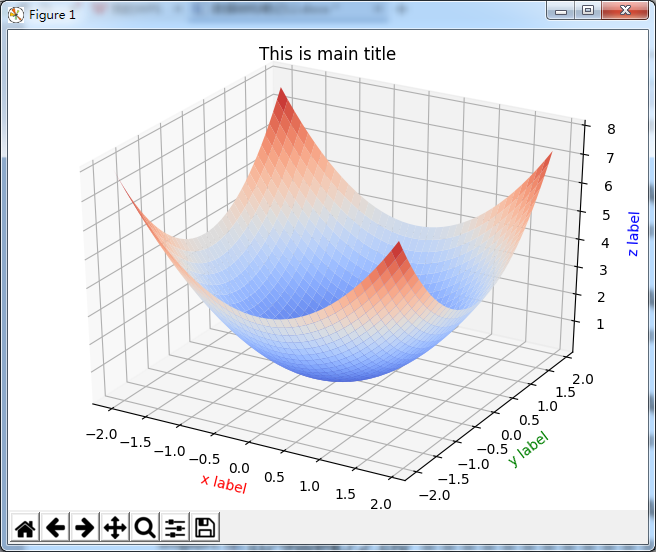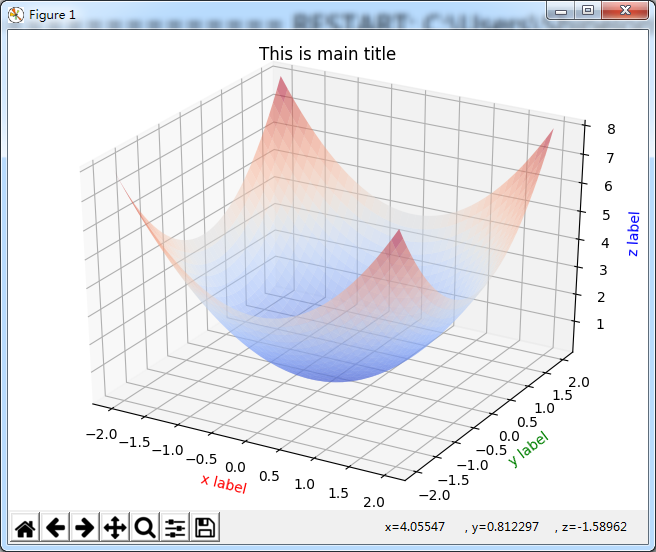# 【python学习笔记】12：用matplotlib绘制3D函数图像

①用pyplot的figure()函数可以创建一个figure对象

②以它为参数创建Axes3D对象，使之具有3D坐标轴

③pyplot的show()方法可以显示所有figure对象

*显示两个3D坐标轴

import matplotlib.pyplot as plt #绘图用的模块
from mpl_toolkits.mplot3d import Axes3D #绘制3D坐标的函数
fig1=plt.figure()#创建一个绘图对象
ax=Axes3D(fig1)#用这个绘图对象创建一个Axes对象(有3D坐标)
fig2=plt.figure()#创建一个绘图对象
ax=Axes3D(fig2)#用这个绘图对象创建一个Axes对象(有3D坐标)
plt.show()#显示模块中的所有绘图对象①用numpy的arange()方法分别创建横坐标，纵坐标可能的取样点值，分别放入两个arange对象中

②用numpy的meshgrid()方法去建立全部的取样点放入之前的两个arange对象中

*建立和扩充取样点横纵坐标

'''import matplotlib.pyplot as plt #绘图用的模块
from mpl_toolkits.mplot3d import Axes3D #绘制3D坐标的函数'''
import numpy as np
'''fig1=plt.figure()#创建一个绘图对象
ax=Axes3D(fig1)#用这个绘图对象创建一个Axes对象(有3D坐标)'''
X=np.arange(-2,2,1)
Y=np.arange(-2,2,1)#创建了从-2到2，步长为1的arange对象
#至此X,Y分别表示了取样点的横纵坐标的可能取值
print ("X为",X)
print ("Y为",Y)
#用这两个arange对象中的可能取值一一映射去扩充为所有可能的取样点
X,Y=np.meshgrid(X,Y)
print ("扩充后X为")
print (X)
print ("扩充后Y为")
print (Y)
'''plt.show()#显示模块中的所有绘图对象'''①用保存取样点横纵坐标的arange对象，传入事先写好的函数，返回给Z，从而为取样点的Z坐标打表

②将三个表传入ax.plot_surface()函数中去用取样点构建曲面，rstride和cstride表示行列隔多少个取样点建一个小面，cmap表示绘制曲面的颜色，在pylot.cm下有很多选项可以选择

*取样点Z坐标打表，按取样点显示曲面

import matplotlib.pyplot as plt #绘图用的模块
from mpl_toolkits.mplot3d import Axes3D #绘制3D坐标的函数
import numpy as np

def fun(x,y):
return np.power(x,2)+np.power(y,2)

fig1=plt.figure()#创建一个绘图对象
ax=Axes3D(fig1)#用这个绘图对象创建一个Axes对象(有3D坐标)
X=np.arange(-2,2,0.1)
Y=np.arange(-2,2,0.1)#创建了从-2到2，步长为0.1的arange对象
#至此X,Y分别表示了取样点的横纵坐标的可能取值
#用这两个arange对象中的可能取值一一映射去扩充为所有可能的取样点
X,Y=np.meshgrid(X,Y)
Z=fun(X,Y)#用取样点横纵坐标去求取样点Z坐标
plt.title("This is main title")#总标题
ax.plot_surface(X, Y, Z, rstride=1, cstride=1, cmap=plt.cm.coolwarm)#用取样点(x,y,z)去构建曲面
ax.set_xlabel('x label', color='r')
ax.set_ylabel('y label', color='g')
ax.set_zlabel('z label', color='b')#给三个坐标轴注明
plt.show()#显示模块中的所有绘图对象X,Y=numpy.mgrid[X的取样下限:X的取样上限:X的分割数j,Y的取样下限:Y的取样上限:Y的分割数j]

*建立和扩充合并做，设置不透明度为0.5

import matplotlib.pyplot as plt #绘图用的模块
from mpl_toolkits.mplot3d import Axes3D #绘制3D坐标的函数
import numpy as np

def fun(x,y):
return np.power(x,2)+np.power(y,2)

fig1=plt.figure()#创建一个绘图对象
ax=Axes3D(fig1)#用这个绘图对象创建一个Axes对象(有3D坐标)
'''X=np.arange(-2,2,0.1)
Y=np.arange(-2,2,0.1)#创建了从-2到2，步长为0.1的arange对象
#至此X,Y分别表示了取样点的横纵坐标的可能取值
#用这两个arange对象中的可能取值一一映射去扩充为所有可能的取样点
X,Y=np.meshgrid(X,Y)'''
X,Y=np.mgrid[-2:2:40j,-2:2:40j]#从-2到2分别生成40个取样坐标，并作满射联合
Z=fun(X,Y)#用取样点横纵坐标去求取样点Z坐标
plt.title("This is main title")#总标题
ax.plot_surface(X, Y, Z, rstride=1, cstride=1, cmap=plt.cm.coolwarm,alpha=0.5)#用取样点(x,y,z)去构建曲面
ax.set_xlabel('x label', color='r')
ax.set_ylabel('y label', color='g')
ax.set_zlabel('z label', color='b')#给三个坐标轴注明
plt.show()#显示模块中的所有绘图对象